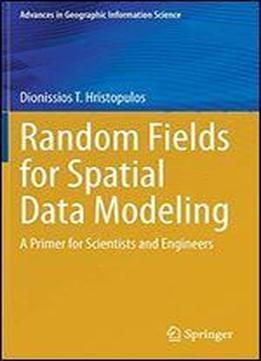# Random Fields For Spatial Data Modeling: A Primer For Scientists And Engineers by Dionissios T. Hristopulos / 2020 / English / PDF

This book provides an inter-disciplinary introduction to the theory of random fields and its applications. Spatial models and spatial data analysis are integral parts of many scientific and engineering disciplines. Random fields provide a general theoretical framework for the development of spatial models and their applications in data analysis. The contents of the book include topics from classical statistics and random field theory (regression models, Gaussian random fields, stationarity, correlation functions) spatial statistics (variogram estimation, model inference, kriging-based prediction) and statistical physics (fractals, Ising model, simulated annealing, maximum entropy, functional integral representations, perturbation and variational methods). The book also explores links between random fields, Gaussian processes and neural networks used in machine learning. Connections with applied mathematics are highlighted by means of models based on stochastic partial differential equations. An interlude on autoregressive time series provides useful lower-dimensional analogies and a connection with the classical linear harmonic oscillator. Other chapters focus on non-Gaussian random fields and stochastic simulation methods. The book also presents results based on the authors research on Spartan random fields that were inspired by statistical field theories originating in physics. The equivalence of the one-dimensional Spartan random field model with the classical, linear, damped harmonic oscillator driven by white noise is highlighted. Ideas with potentially significant computational gains for the processing of big spatial data are presented and discussed. The final chapter concludes with a description of the Karhunen-Love expansion of the Spartan model. The book will appeal to engineers, physicists, and geoscientists whose research involves spatial models or spatial data analysis. Anyone with background in probability and statistics can read at least parts of the book. Some chapters will be easier to understand by readers familiar with differential equations and Fourier transforms.

views: 158